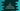# 4 different ways to print the natural numbers in reverse order in C## How to print the natural numbers in reverse order in C:

In this post, we will learn how to print the natural numbers in reverse order in C programming. We will write one program that will take a number as input from the user and print the numbers in reverse order starting from that number. It will stop at 1.

We can use any loop to write this program. In this post, I will show you how to use a for loop, while loop, and a do while loop to write the same program.

### Method 1: C program to print the natural numbers in reverse by using a for loop:

Let’s learn how we can use a for loop to print the natural numbers in reverse in C.

``````#include <stdio.h>

void printReverse(int n)
{
for (int i = n; i > 0; i--)
{
printf("%d ", i);
}
}

int main()
{
int n;

printf("Enter the value of n: ");
scanf("%d", &n);

printf("Natural numbers from %d to 1:\n", n);
printReverse(n);
printf("\n");
}``````

Here,

• It asks the user to enter the value of n. It uses scanf to read the value and stores it in the integer variable n.
• The printReverse method is used to print the numbers from n to 1.
• Inside printReverse, we are using a for loop to print the values. This loop runs from i = n and it will keep running until the value of i is greater than 0. On each iteration, we are decrementing the value of i by 1.
• Inside the loop, we are printing the value of i.

If you run this program, it will print output as like below:

``````Enter the value of n: 10
Natural numbers from 10 to 1:
10 9 8 7 6 5 4 3 2 1``````

### Method 2: By using a while loop:

Let’s write down the same program by using a while loop. We can change the printReverse method to replace the for loop with a while loop.

``````#include <stdio.h>

void printReverse(int n)
{
int i = n;
while (i > 0)
{
printf("%d ", i);
i--;
}
}

int main()
{
int n;

printf("Enter the value of n: ");
scanf("%d", &n);

printf("Natural numbers from %d to 1:\n", n);
printReverse(n);
printf("\n");
}``````

It is similar to the first program. We are using a while loop and this loop will run until the value of i is greater than 0. The starting value of i is n and on each iteration it’s value is decremented by 1. Inside the loop, we are printing the current value of i.

It will print similar result.

### Method 3: By using a do-while loop:

The while loop checks the condition and runs the code if the condition is true. But, do-while loop runs the code of the do block and then it checks the condition. If we want to use a do-while loop to print the natural numbers in reverse, we can do it as like below:

``````#include <stdio.h>

void printReverse(int n)
{
int i = n;
do
{
printf("%d ", i);
i--;
} while (i > 0);
}

int main()
{
int n;

printf("Enter the value of n: ");
scanf("%d", &n);

printf("Natural numbers from %d to 1:\n", n);
printReverse(n);
printf("\n");
}``````

It will give similar result.

### Method 4: Print all natural numbers in a range:

We can also change the above programs to print all natural numbers in a range i.e. it will start at one value and end at another value instead of 1. Let’s write down this program:

``````#include <stdio.h>

void printReverse(int n, int j)
{
for (int i = n; i >= j; i--)
{
printf("%d ", i);
}
}

int main()
{
int n, j;

printf("Enter the value value of n: ");
scanf("%d", &n);

printf("Enter the value to stop: ");
scanf("%d", &j);

printf("Natural numbers from %d to %d:\n", n, j);
printReverse(n, j);
printf("\n");
}``````

Here,

• It takes two values from the user.
• The printReverse method is changed and it takes two parameters, n and j.
• The for loop runs from i = n to j and it prints the value of i inside the loop body.

If you run this program, it will give output as like below:

``````Enter the value value of n: 100
Enter the value to stop: 81
Natural numbers from 100 to 81:
100 99 98 97 96 95 94 93 92 91 90 89 88 87 86 85 84 83 82 81``````

We can also write the same program with any other loop.